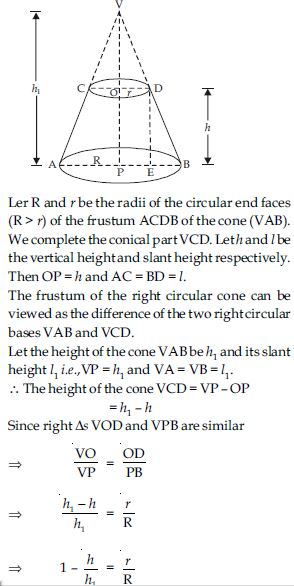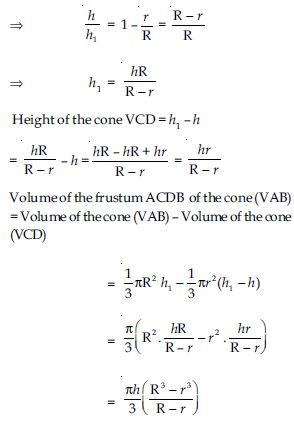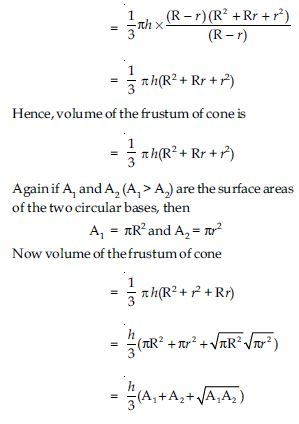# NCERT Solutions for Class 10 Math Chapter 13 - Surface Areas and Volumes

Aasoka has covered all the questions of the chapter “Surface Areas and Volumes” in the NCERT Solutions for Class 10th. Students can get free access to the solutions which are beneficial for the exam preparations. The NCERT Solutions helps in efficiently revising the entire chapter as it is designed following the latest syllabus. Students can put their trust in Aasoka’s top-quality study material to get started with their board exam preparation.

In the chapter “Surface Areas and Volumes” of NCERT Class 10th Math, students will learn about surface area and volumes of various shapes and objects. In the exercises included in the chapter, the question includes converting shape from one form to another, finding the volume, total surface area of shapes, curved surface area, etc.

##### Question 1:

2 cubes each of volume 64 cm3 are joined end to end. Find the surface area of the resulting cuboid.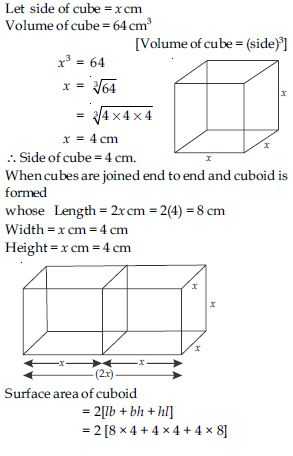= 2 [32 + 16 + 32] = 2 
∴ Surface area of cuboid = 160 cm2.

##### Question 2:

A vessel is in the form of a hollow semisphere mounted by a hollow cylinder. The diameter of the hemisphere is 14 cm and the total height of the vessel is 13 cm. Find the inner surface area of the vessel.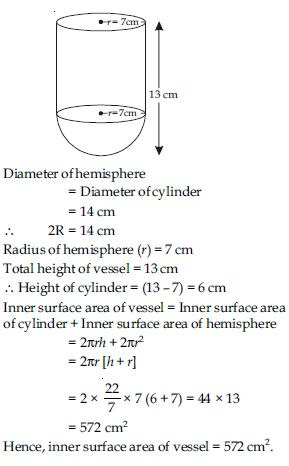##### Question 3:

A toy is in the form of a cone of radius 3.5 cm mounted on a hemisphere of same radius. The total height of the toy is 15.5 cm. Find the total surface area of the toy.

= 3.5 cm
Total height of toy = 15.5 cm
∴ Height of cone (h) = (15.5 – 3.5) cm = 12 cm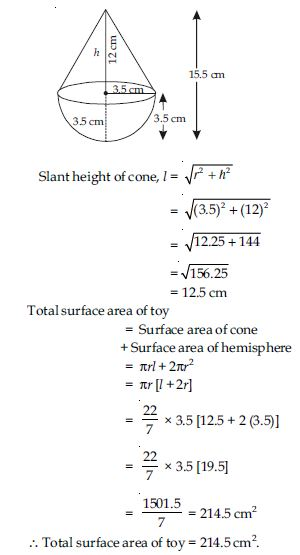##### Question 4:

A cubical block of side 7 cm is surmounted by a hemisphere. What is the greatest diameter the hemisphere can have? Find the surface area of the solid.

Side of cubical box = a = 7 cm
Diameter of hemisphere
= Side of cubical box = 7 cm
2r = 7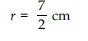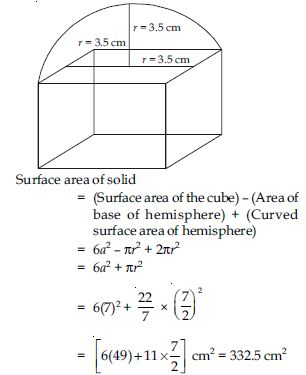##### Question 5:

A hemispherical depression is cut out from one face of a cubical wooden block such that the diameter l of the hemisphere is equal to the edge of the cube. Determine the surface area of the remaining solid.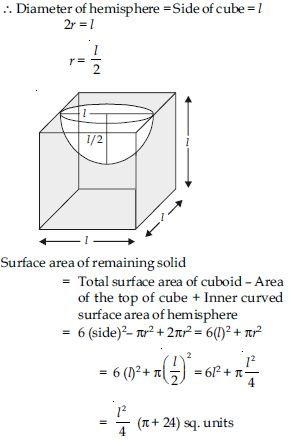##### Question 6:

A medicine capsule is in the shape of a cylinder with two hemispheres stuck to each of its ends. The length of the entire capsule is 14 mm and the diameter of the capsule is 5 mm. Find its surface area.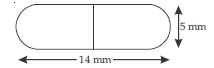Diameter of capsule
= Diameter of hemisphere
= Diameter of cylinder = 5 mm
∴ 2r = 5 mm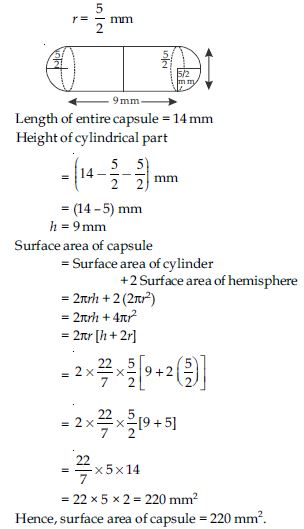##### Question 7:

A tent is in the shape of a cylinder surmounted by a conical top. If the height and diameter of the cylindrical part are 2.1 m and 4 m respectively, and the slant height of the top is 2.8 m, find the area of the canvas used for making the tent. Also, find the cost of the canvas of the tent at the rate of ₹500 per m2. (Note that the base of the tent will not be covered with canvas.)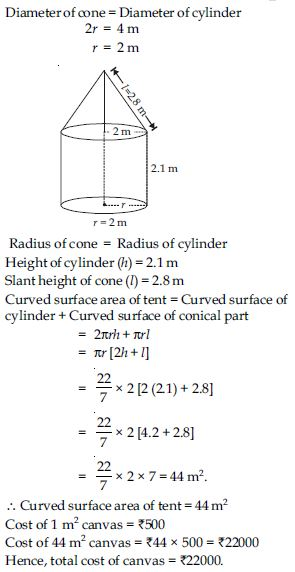##### Question 8:

From a solid cylinder whose height is 2.4 cm and diameter 1.4 cm, a conical cavity of the same height and same diameter is hollowed out. Find the total surface area of the remaining solid to the nearest cm2.

Diameter of cylinder (D) = 1.4 cm
= Diameter of cone
= 0.7 cm
Height of cylinder (h) = 2.4 cm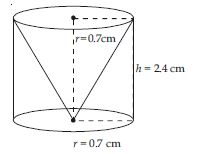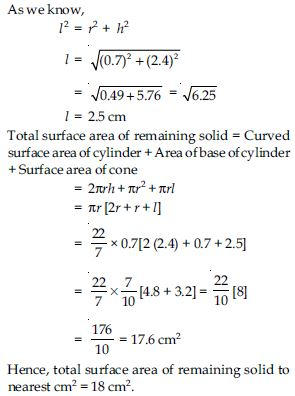##### Question 9:

A wooden article was made by scooping out a hemisphere from each end of a solid cylinder, as shown in fig.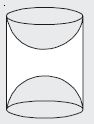If the height of the cylinder is 10 cm, and its base is of radius 3.5 cm, find the total surface area of the article.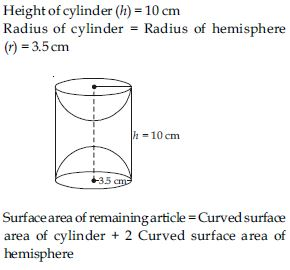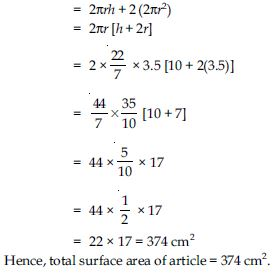##### Question 10:

A solid is in the shape of a cone standing on a hemisphere with both their radii being equal to 1 cm and the height of the cone is equal to its radius. Find the volume of the solid in terms of $\mathrm{\pi }$.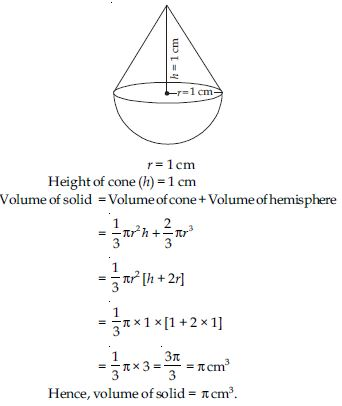##### Question 11:

Rachel, an engineering student, was asked to make a model shaped like a cylinder with two cones attached at its two ends by using a thin aluminium sheet. The diameter of the model is 3 cm and its length is 12 cm. If each cone has a height of 2 cm, find the volume of air contained in the model that Rachel made. (Assume the outer and inner dimensions of the model to be nearly the same.)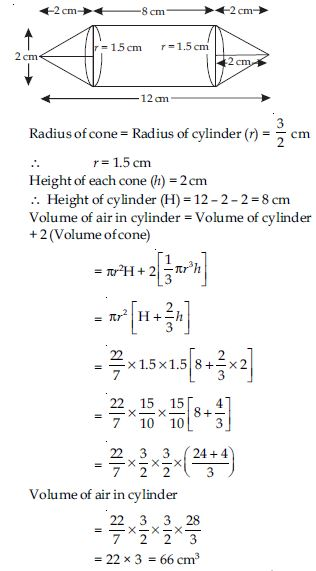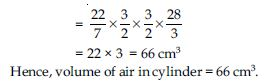##### Question 12:

A gulab jamun, contains sugar syrup up to about 30% of its volume. Find approximately how much syrup would be found in 45 gulab jamuns, each shaped like a cylinder with two hemispherical ends with length 5 cm and diameter 2.8 cm.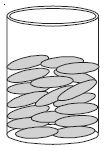Gulab jamun is in the shape of cylinder.
Diameter of cylinder = Diameter of hemisphere
= 2.8 cm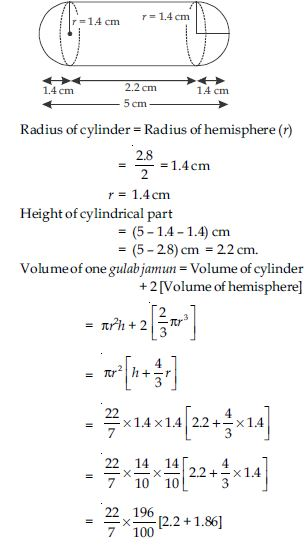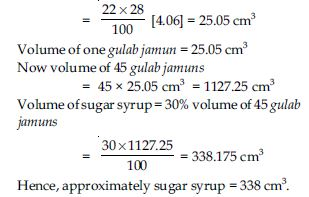##### Question 13:

A pen stand made of wood is in the shape of a cuboid with four conical depressions to hold pens. The dimensions of the cuboid are 15 cm by 10 cm by 3.5 cm. The radius of each of the depressions is 0.5 cm and the depth is 1.4 cm. Find the volume of wood in the entire stand.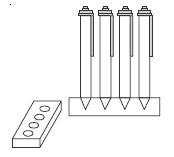Length of cuboid (l) = 15 cm
Width of cuboid (b) = 10 cm
Height of cuboid (h) = 3.5 cm
Radius of conical cavity (r) = 0.5 cm
Height of conical cavity (h) = 1.4 cm
Volume of wood in pen stand = Volume of
cuboid – 4 [Volume of cone]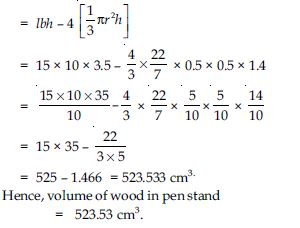##### Question 14:

A vessel is in the form of an inverted cone. Its height is 8 cm and the radius of its top, which is open, is 5 cm. It is filled with water up to the brim. When lead shots, each of which is a sphere of radius 0.5 cm are dropped into the vessel, one-fourth of the water flows out. Find the number of lead shots dropped in the vessel.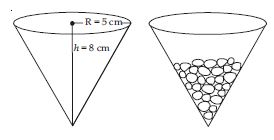Radius of cone (R) = 5 cm
Height of cone (h) = 8 cm
Let number of shots put into the cone = N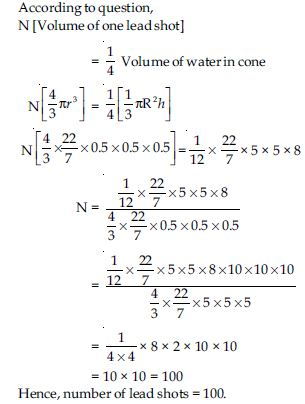##### Question 15:

A solid iron pole consists of a cylinder of height 220 cm and base diameter 24 cm, which is surmounted by another cylinder of height 60 cm and radius 8 cm. Find the mass of the pole, given that 1 cm3 of iron has approximately 8 g mass. (Use $\mathrm{\pi }$ = 3.14)

Diameter of lower cylinder = 24 cm
Radius of lower cylinder (R) = 12 cm
Height of lower cylinder (H) = 220 cm
Radius of upper cylinder (r) = 8 cm
Height of upper cylinder (h) = 60 cm
Volume of pole = Volume of lower cylinder +
Volume of upper cylinder
= $\mathrm{\pi }$R2H + $\mathrm{\pi }$r2h
= 3.14 × 12 × 12 × 220 + 3.14 × 8 × 8 × 60
= 99475.2 + 12057.6 = 111532.8 cm3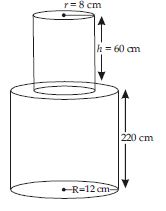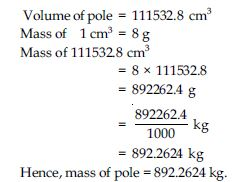##### Question 16:

A solid consisting of a right circular cone of height 120 cm and radius 60 cm standing on a hemisphere of radius 60 cm is placed upright in a right circular cylinder full of water such that it touches the bottom. Find the volume of water left in the cylinder, if the radius of the cylinder is 60 cm and its height is 180 cm.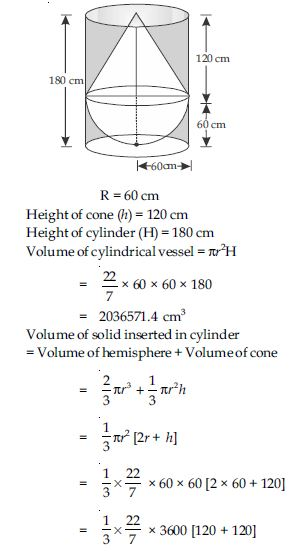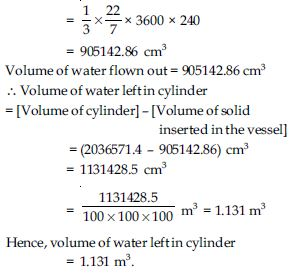##### Question 17:

A spherical glass vessel has a cylindrical neck 8 cm long, 2 cm in diameter; the diameter of the spherical part is 8.5 cm. By measuring the amount of water it holds, a child finds its volume to be 345 cm3. Check whether she is correct, taking the above as the inside measurements, and $\mathrm{\pi }$ = 3.14.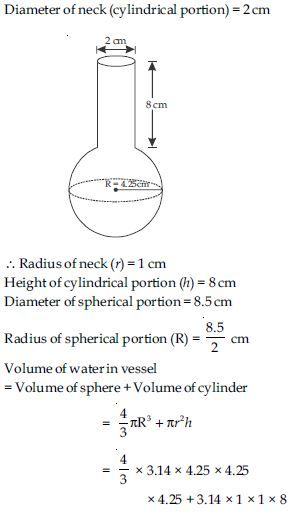= 321.39 + 25.12 = 346.51 cm3
Hence, volume of water in vessel = 346.51 cm3 and she is wrong.

##### Question 18:

A metallic sphere of radius 4.2 cm is melted and recast into the shape of cylinder of radius 6 cm. Find the height of the cylinder.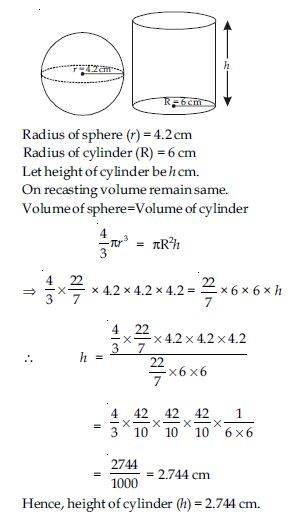##### Question 19:

Metallic spheres of radii 6 cm, 8 cm and 10 cm, respectively, are melted to form a single solid sphere. Find the radius of the resulting sphere.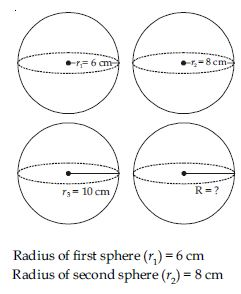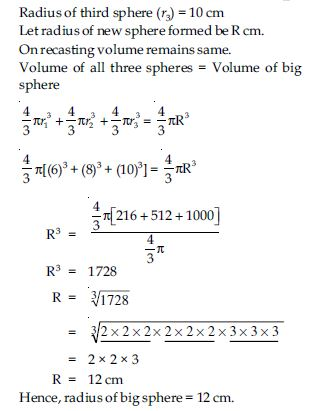##### Question 20:

A 20 m deep well with diameter 7 m is dug and the earth from digging is evenly spread out to form a platform 22 m by 14 m. Find the height of the platform.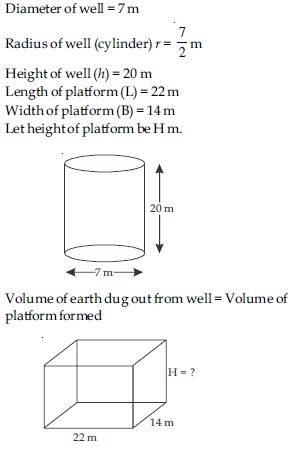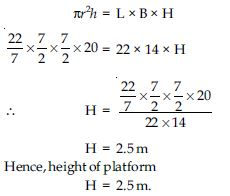##### Question 21:

A well of diameter 3 m is dug 14 m deep. The earth taken out of it has been spread evenly all around it in the shape of a circular ring of width 4 m to form an embankment. Find the height of the embankment.

Depth of well (h) = 14 m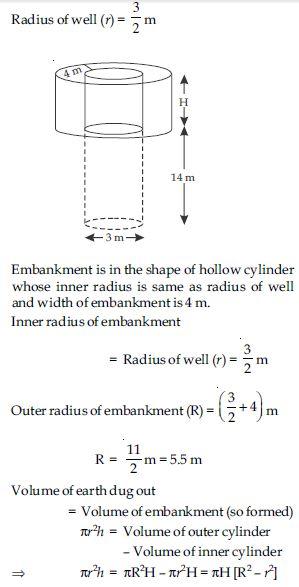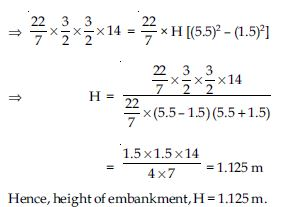##### Question 22:

A container shaped like a right circular cylinder having diameter 12 cm and height 15 cm is full of ice cream. The ice cream is to be filled into cones of height 12 cm and diameter 6 cm, having a hemispherical shape on the top. Find the number of such cones which can be filled with ice cream.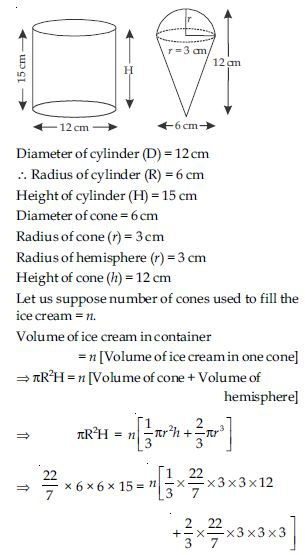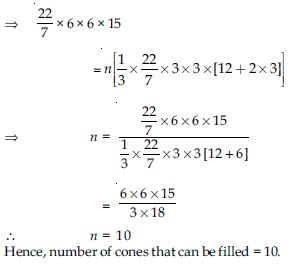##### Question 23:

How many silver coins, 1.75 cm in diameter and of thickness 2 mm, must be melted to form a cuboid of dimensions 5.5 cm × 10 cm × 3.5 cm?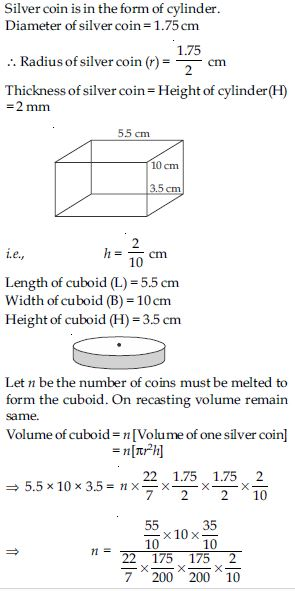n = 400
Hence, number of coins to be melted = 400.

##### Question 24:

A cylindrical bucket, 32 cm high and with radius of base 18 cm, is filled with sand. This bucket is emptied on the ground and a conical heap of sand is formed. If the height of the conical heap is 24 cm, find the radius and slant height of the heap.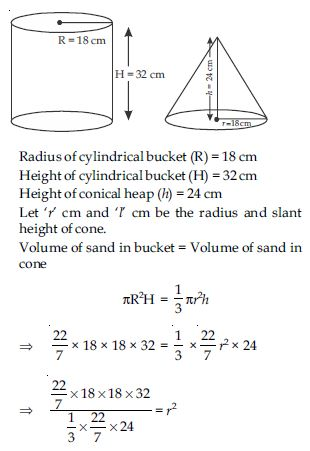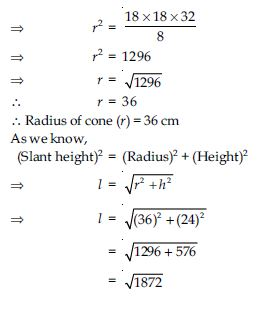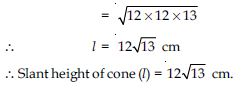##### Question 25:

Water in a canal 6 m wide and 1.5 m deep is flowing with a speed of 10 km/h. How much area will it irrigate in 30 minutes, if 8 cm of standing water is needed?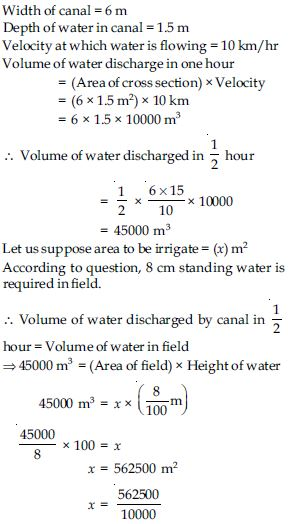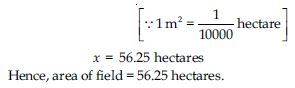##### Question 26:

A farmer connects a pipe of internal diameter 20 cm from a canal into a cylindrical tank in her field, which is 10 m in diameter and 2 m deep. If water flows through the pipe at the rate of 3 km/h, in how much time will the tank be filled?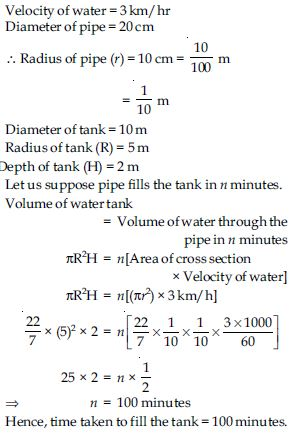##### Question 27:

A drinking glass is in the shape of a frustum of a cone of height 14 cm. The diameters of its two circular ends are 4 cm and 2 cm. Find the capacity of the glass.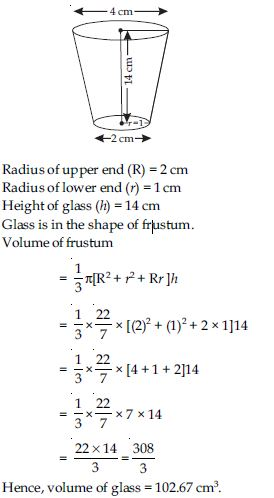##### Question 28:

The slant height of a frustum of a cone is 4 cm and the perimeters (circumference) of its circular ends are 18 cm and 6 cm. Find the curved surface area of the frustum.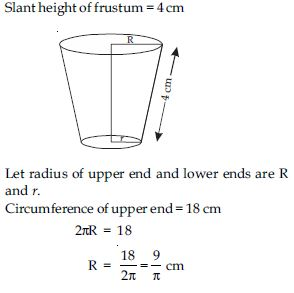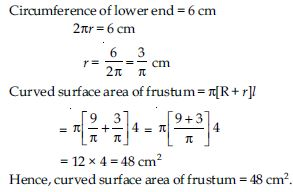##### Question 29:

A fez, the cap used by the Turks, is shaped like the frustum of a cone. If its radius on the open side is 10 cm, radius at the upper base is 4 cm and its slant height is 15 cm, find the area of material used for making it.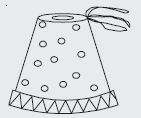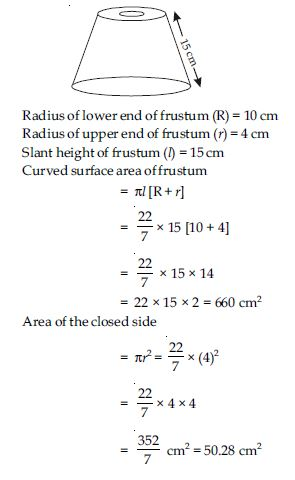Total area of the material used = Curved surface
area of frustum + Area of the closed side
= 660 + 50.28 = 710.28 cm2
Hence, the area of total material used = 710.28 cm2.

##### Question 30:

A container opened from the top is made up of a metal sheet is in the form of a frustum of a cone of height 16 cm with radii of its lower and upper ends as 8 cm and 20 cm, respectively. Find the cost of the milk which can completely fill the container, at the rate, of ₹20 per litre. Also find the cost of metal sheet used to make the container, if it costs ₹8 per 100 cm2. (Take $\mathrm{\pi }$ = 3.14.)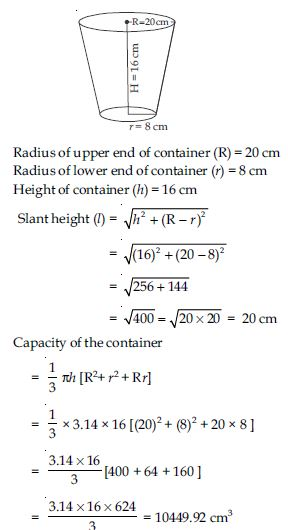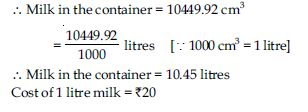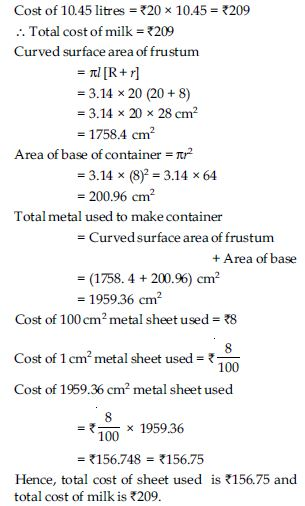##### Question 31: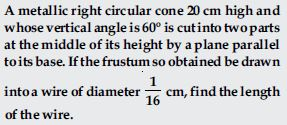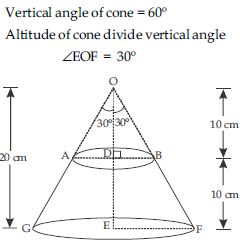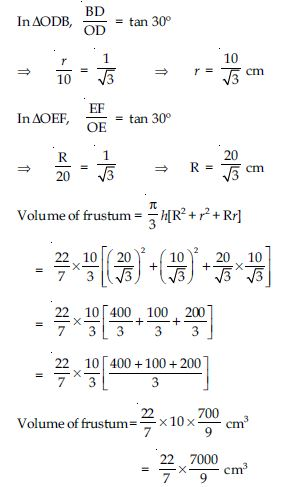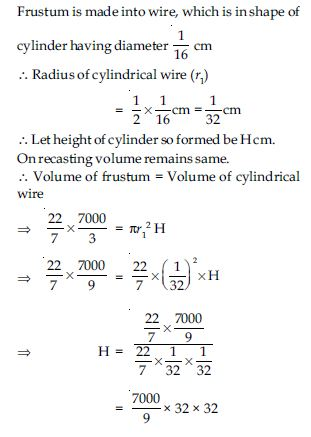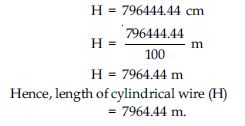##### Question 32:

A copper wire 3 mm in diameter is wound about a cylinder whose length is 12 cm, and diameter 10 cm, so as to cover the curved surface of the cylinder. Find the length and mass of the wire, assuming the density of copper to 8.88 g per cm3.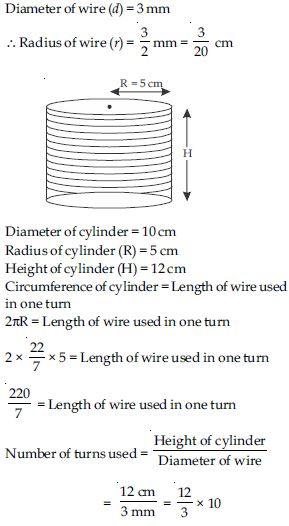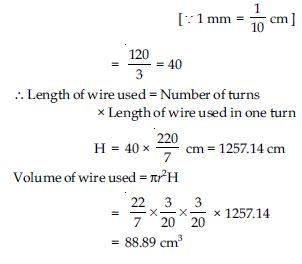Mass of 1 cm3 = 8.88 g
Mass of 88.89 cm3 = 8.88 × 88.89 = 789.41 g
Hence, length of wire is 1257.14 cm and mass of wire is 789.41 g.

##### Question 33:

A right triangle, whose sides are 3 cm and 4 cm (other than hypotenuse) is made to revolve about its hypotenuse. Find the volume and surface area of the double cone so formed. (Choose value of $\mathrm{\pi }$ as found appropriate.)

Let $∆$ABC be the right triangle right angled at A whose sides AB and AC measure 3 cm and 4 cm respectively.
The length of the side BC (hypotenuse)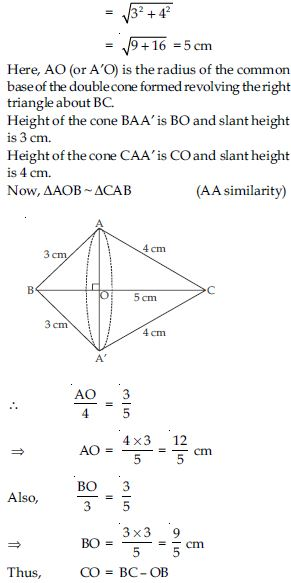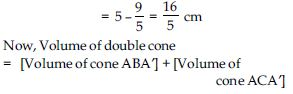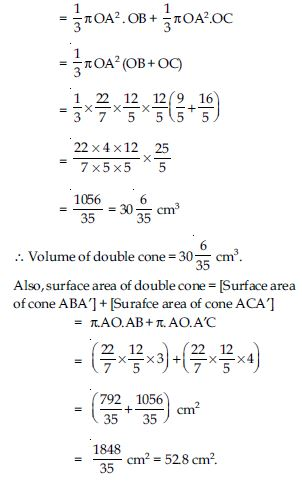##### Question 34:

A cistern, internally measuring 150 cm × 120 cm × 110 cm, has 129600 cm3 of water in it. Porous bricks are placed in the water until the cistern is full to the brim. Each brick absorbs one-seventeenth of its own volume of water. How many bricks can be put in without overflowing of water, each brick being 22.5 cm × 7.5 cm × 6.5 cm?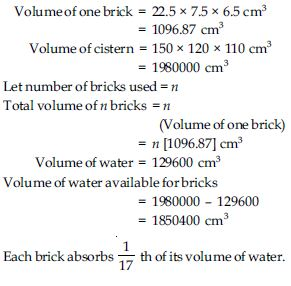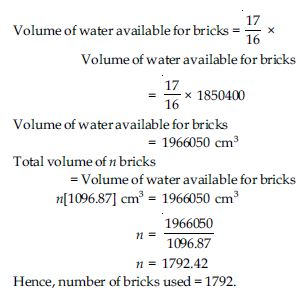##### Question 35:

In one fortnight of a given month, there was a rainfall of 10 cm in a river valley. If the area of the valley is 7280 km2, show that the total rainfall was approximately equivalent to the addition to the normal water of three rivers each 1072 km long, 75 m wide and 3 m deep.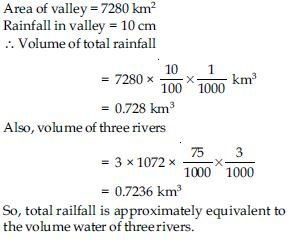##### Question 36:

An oil funnel made of tin sheet consists of a 10 cm long cylindrical portion attached to a frustum of a cone. If the total height is 22 cm, diameter of the cylindrical portion is 8 cm and the diameter of the top of the funnel is 18 cm, find the area of the tin sheet required to make the funnel.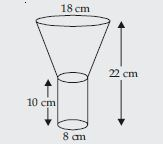##### Question 37:

Derive the formula for the curved surface area and total surface area of a frustum of a cone, given to you in Section 13.5, using the symbols as explained.

A frustum of a right circular cone has two unequal flat circular bases and a curved surface. Let ACDB be the frustum of the cone obtained by removing the portion VCD. The line-segment OP joining the centres of two bases is called the height of the frustum. Each of the line-segments AC and BD of frustum ACDB is called its slant height.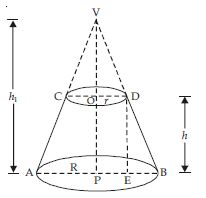Let R and r be the radii of the circular end faces (R > r) of the frustum ACDB of the cone (VAB). We complete the conical part VCD. Let h and l be the vertical height and slant height respectively. Then OP = h and AC = BD = l.
The frustum of the right circular cone can be viewed as the difference of the two right circular bases VAB and VCD.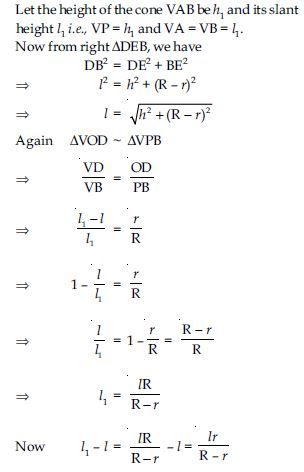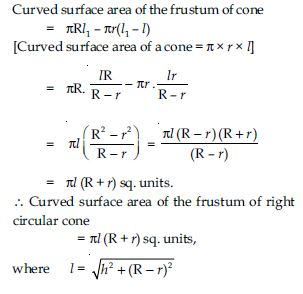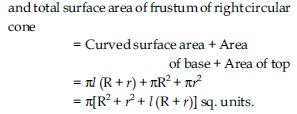##### Question 38:

Derive the formula for the volume of the frustum of a cone, given to you in Section 13.5, using the symbols as explained.